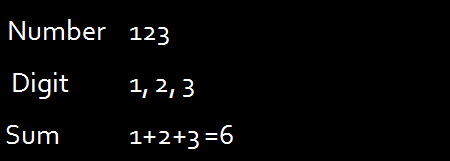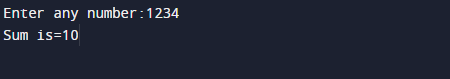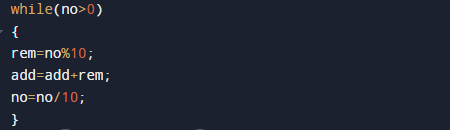C Program to Calculate Sum Of Digits In a Number

In this tutorial you will learn about the C Program to Calculate Sum Of Digits In a Number and its application with practical example.

In this tutorial, we will learn to create a C program that will find sum of inputted values  using C programming.

Prerequisites

Before starting with this tutorial we assume that you are best aware of the following C programming topics:

• C Operators.
• Basic input/output.
• C loop statements.
• C for Loop.

Example

C program to calculate the sum of digits of any number inputted by user, here we use modulus operator (%) to break digits of a number and keep on adding them.you can see what we gonna do in our example.Logic to find sum of digits of a number

The idea behind  to find sum of digits is.

suppose number is 21.

Break the last digit of the given number.      //=> step=>  no%21=>1=>rem=1

2. By removing last digit from given number.  // =>  no=21/20 => no=2.

Must repeat above steps till the number became 0. Finally you will get sum of digits.

Program for Sum of Digits of a Given Number.

In this program we will find the sum of digit of a given number  using while loop.
Firstly we declare required header file and variable and also initiates required values in variable. Next we take value from user at run time and then after we will find the sum of digit using following logic.

OutputIn the above program, we have first declared and initialized a set variables required in the program.

• no = number inputted by user.
• rem=hold remainder in between.

Sum of digits Steps.

To get sum of each digits by c program, use the following steps.

• Step 1: Take  any number from user.
• Step 2: break last number of digit and store in rem. (rem=no%10)
•